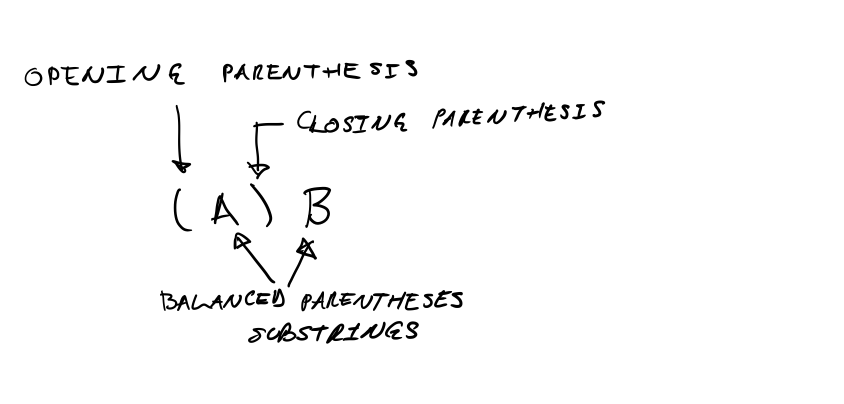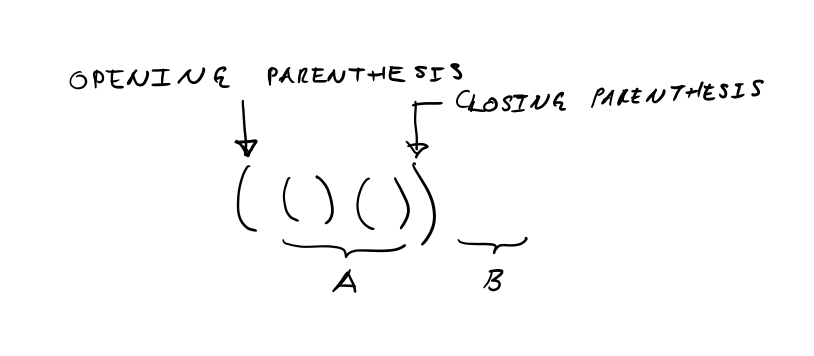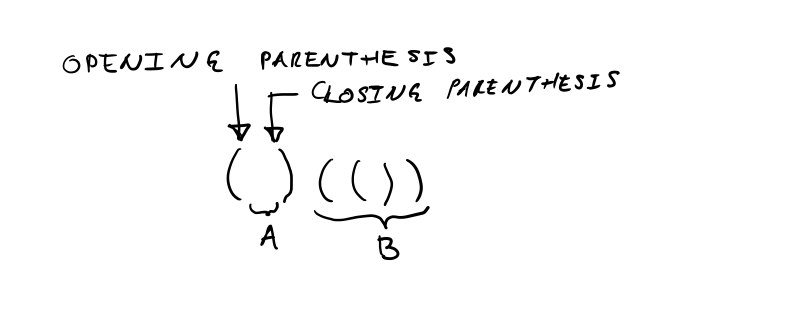We explain another approach to solving All Balanced Parentheses Strings and implement the approach in Ruby and Golang.

If you haven’t read All Balanced Parentheses Strings, do it now.

# Solution

The structure of any given string consisting of n balanced pairs of parentheses falls in one of the following two cases.

Case n = 0. The empty string is the only balanced parentheses string that has no pair of parentheses.

Case n > 0. The following diagram illustrates the structure of the given string.For example, consider string (()()).The string begins with an opening parenthesis and contains a corresponding closing parenthesis. The example contains substring ()() between the opening and closing parentheses, which corresponds to A in the previous diagram. The example does not have a substring after the closing parenthesis, so the empty string corresponds to B in the previous diagram.

As another example, consider string ()(()).In this example, the empty string corresponds to A in the previous diagram and substring (()) corresponds to B.

Each example satisfies the condition that the sum of balanced pairs of parentheses in A and B is one less than the count of balanced pairs of parentheses in the whole string.

Our approach to enumerating all strings for a given n consists in recursively applying the two cases to the construction of all pairs of substrings A and B that satisfy condition pairs(A) + pairs(B) = n - 1. For example, for the construction of all strings where n = 3, we construct the strings for n = 2, 1, 0, and concatenate all pairs that satisfy the condition with opening and closing parentheses, like so.

Our application of the two cases corresponds to the application of the following recursive definition of the set $e_n$ of strings consisting of n balanced pairs of parentheses.

$e_0 = \{ “” \}$

$e_n = \{ “\text{(}A\text{)}B” \mid \exists i, j : A \in e_i \wedge B \in e_j \wedge i + j = n - 1 \}$

# Implementation

We implement the solution in Ruby and Golang.

Our Ruby implementation is the following.

Our Golang implementation is the following.